Geometry 9-3 Rotations
starstarstarstarstarstarstarstarstarstar
by Matthew Richardson
| 28 Questions
Note from the author:
A complete formative lesson with embedded slideshow, mini lecture screencasts, checks for understanding, practice items, mixed review, and reflection. I create these assignments to supplement each lesson of Pearson's Common Core Edition Algebra 1, Algebra 2, and Geometry courses. See also mathquest.net and twitter.com/mathquestEDU.1
2
3
4
1
5
Solve It! In the diagram, the point (3, 2) is rotated counterclockwise about the origin. The point (x₁, y₁) is the result of a 90° rotation. What are the coordinates of (x₁, y₁)?
(-3, -2)
(3, -2)
(2, -3)
(-2, 3)
2
5
Solve It! The point (x₂, y₂) is the result of a 180° rotation. What are the coordinates of (x₂, y₂)? Enter only the coordinates in your response. Include a space after the comma.
3
5
Solve It! The point (x₃, y₃) is the result of a 270° rotation. What are the coordinates of (x₃, y₃)? Enter only the coordinates in your response. Include a space after the comma.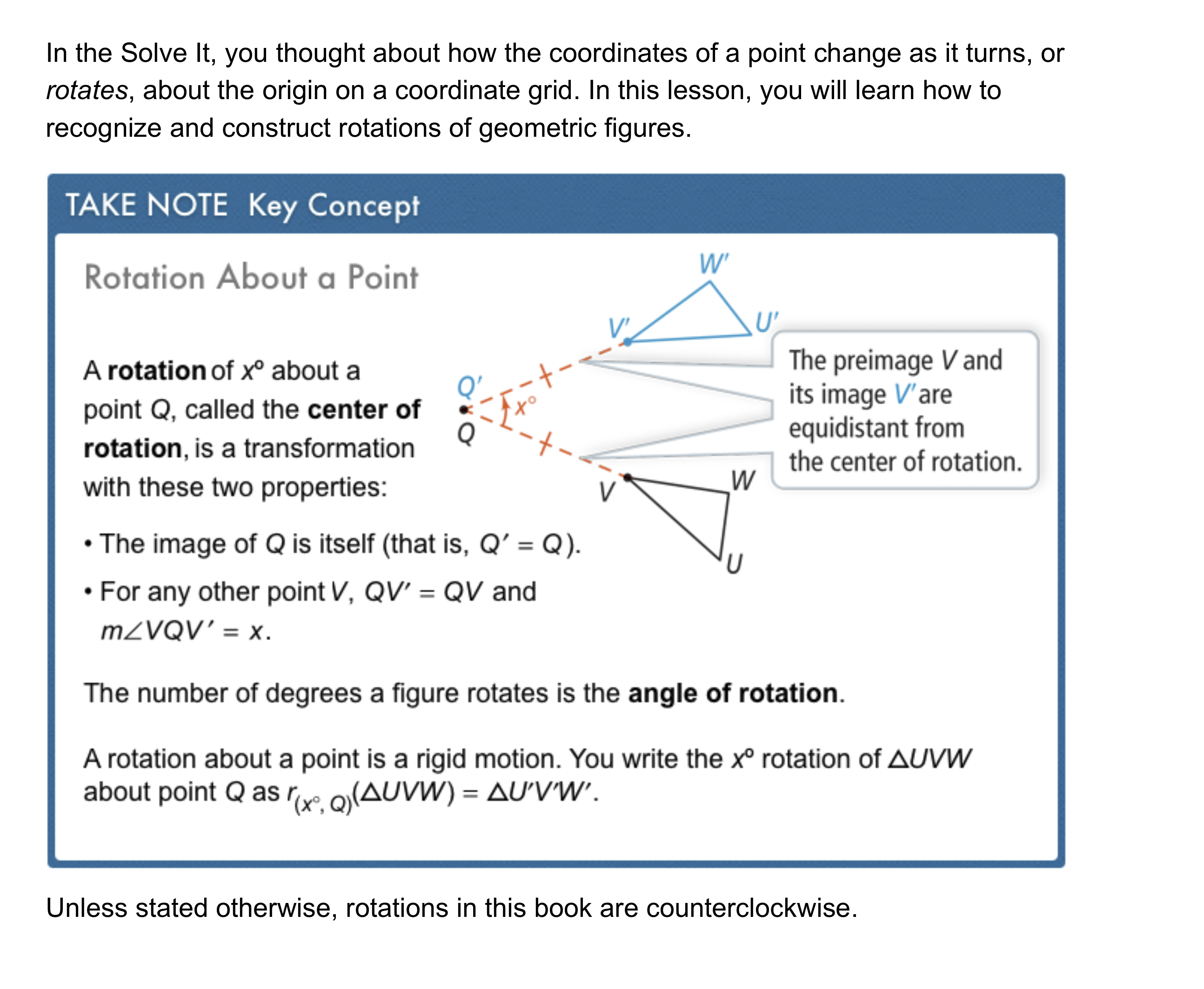5
10
Problem 1 Got It? Draw the image of △LOB for a 50° rotation about B. Use blue or green for the image and label L', O', and B'.6
10
Problem 2 Got It? Graph the rotation on the canvas.7
7
20
Problem 3 Got It? Can you use the properties of rotations to prove that WXYZ is a rhombus? Explain.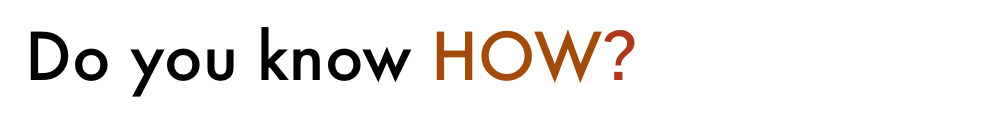8
10
Graphing: Draw the indicated rotation of △ABC on the canvas. Use blue or green for the image and label A', B', and C'.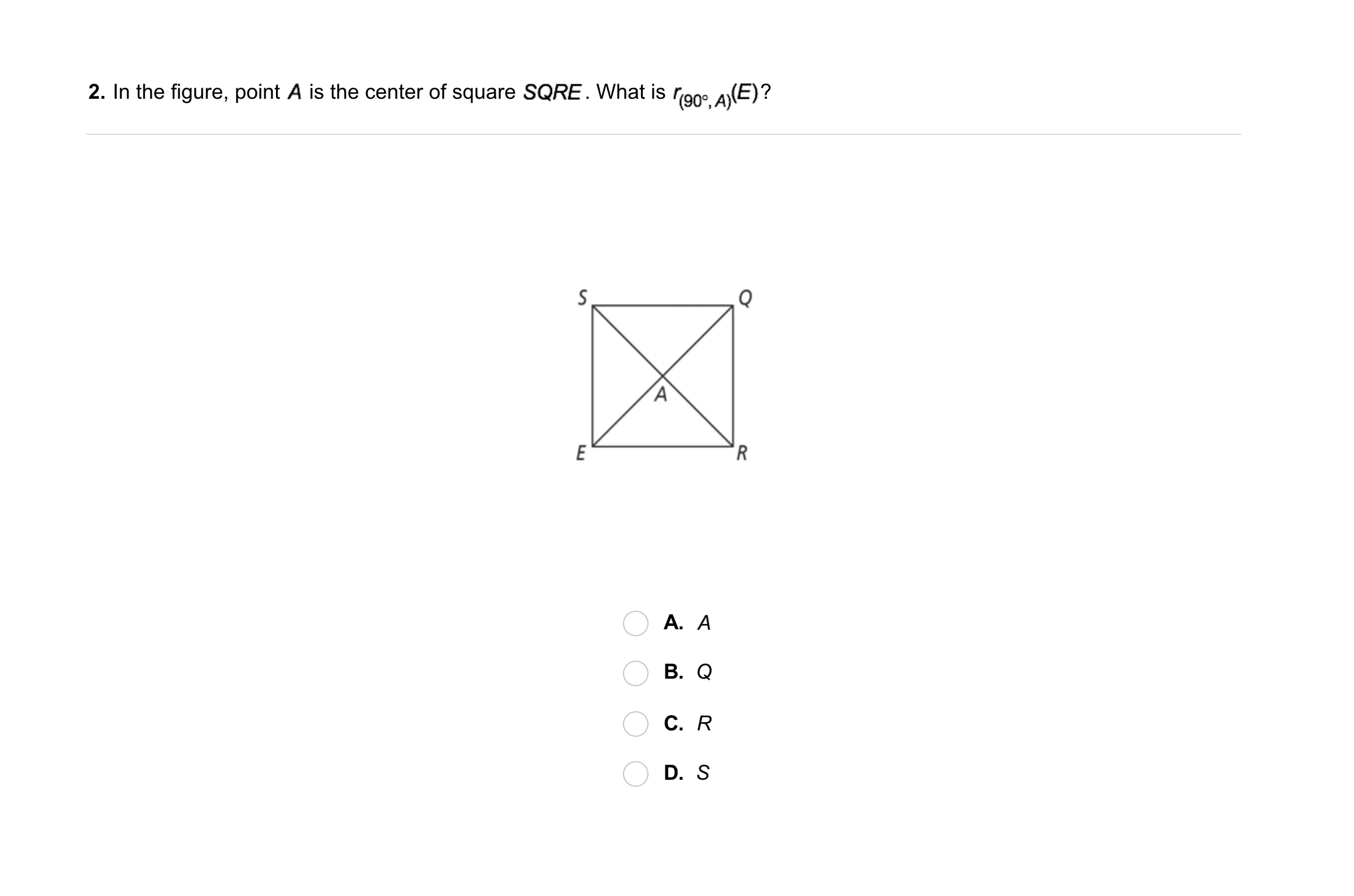9
9
10
A
B
C
D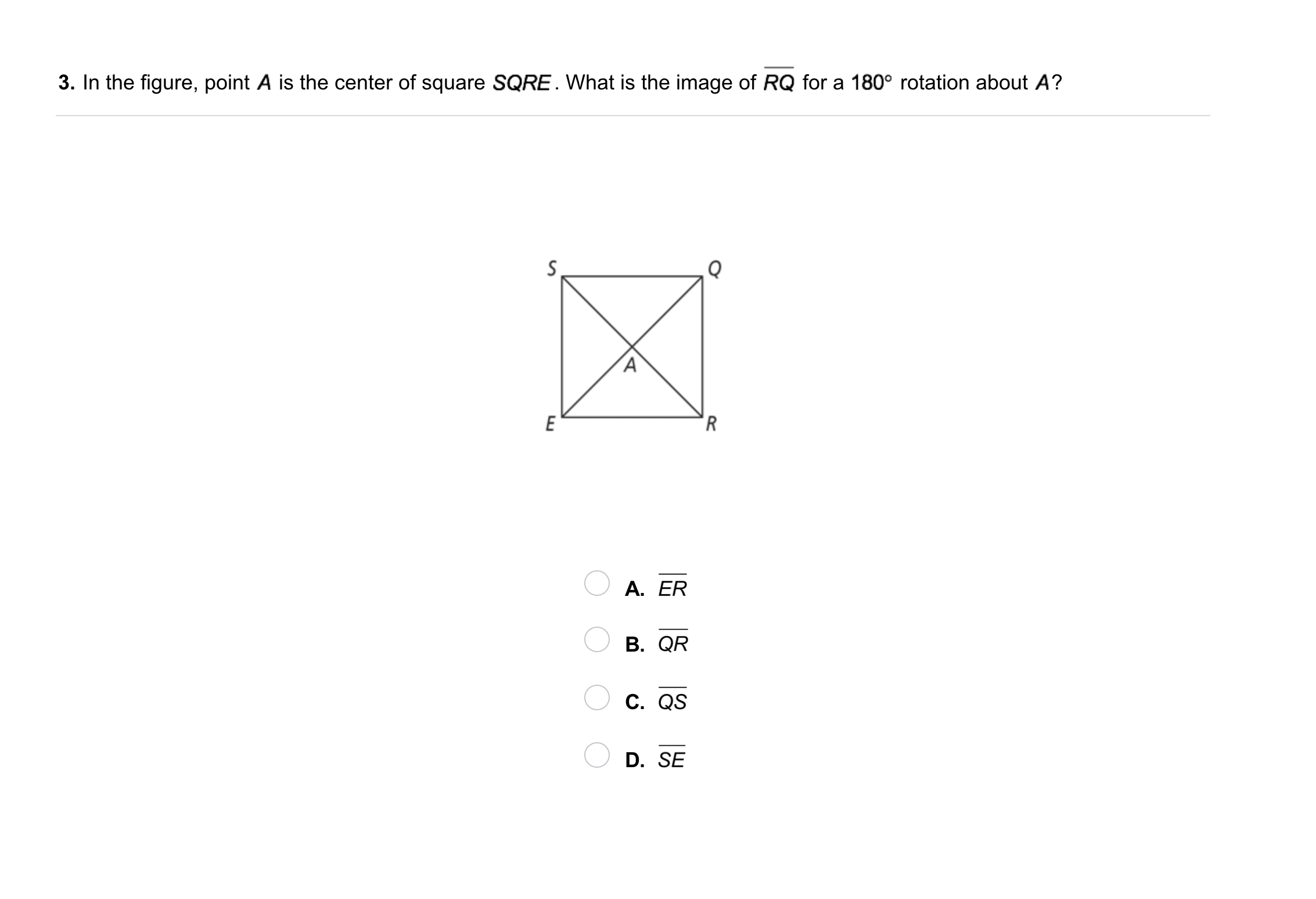10
10
10
A
B
C
D11
10
Vocabulary:A'B'C' is a rotation of △ABC about point O. Describe how to find the angle of rotation.12
12
10
Error Analysis: A classmate drew a 115° rotation of △PQR about point P, as shown. Explain your classmate's error.
13
10
Compare and Contrast: Compare rotating a figure about a point to reflecting the figure across a line. How are the transformations alike? How are they different?
14
10
Reasoning: Point P(x, y) is rotated about the origin by 135° and then by 45°. What are the coordinates of the image of point P? Explain.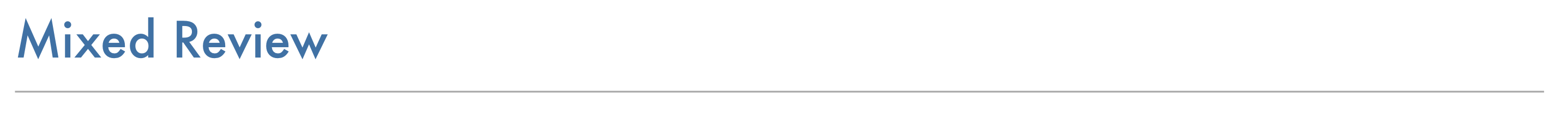15
10
Review Lesson 9-2:BIG has vertices B(-4, 2), I(0, -3), and G(1, 0). Graph △BIG in black and its reflection across the y-axis in green or blue. Label all vertices.
16
10
Review Lesson 9-2:BIG has vertices B(-4, 2), I(0, -3), and G(1, 0). Graph △BIG in black and its reflection across the x-axis in green or blue. Label all vertices.
17
10
Review Lesson 9-2:BIG has vertices B(-4, 2), I(0, -3), and G(1, 0). Graph △BIG in black and its reflection across the line x = 2 in green or blue. Label all vertices.18
19
18
10
Review Lessons 8-2 & 8-3: Find the value of x. Round your answer to the nearest tenth and include units in the same format shown in the diagram.
19
10
Review Lessons 8-2 & 8-3: Find the value of x. Round your answer to the nearest tenth and include units in the same format shown in the diagram.
20
10
Review Lessons 9-2 & 9-3: What are the coordinates of the image of point A(-2, 3) after two 90° rotations about the origin? Enter only the coordinates and include a space after the comma.
21
10
Review Lessons 9-2 & 9-3: What are the coordinates of the image of point T(3, 0) after a reflection across the y-axis followed by a 180° rotation about the origin? Enter only the coordinates and include a space after the comma.
22
10
Review Lessons 9-2 & 9-3: The image of point H after a 90° rotation about the origin followed by a reflection across the x-axis is K(3, 2). What are the coordinates of H? Enter only the coordinates and include a space after the comma.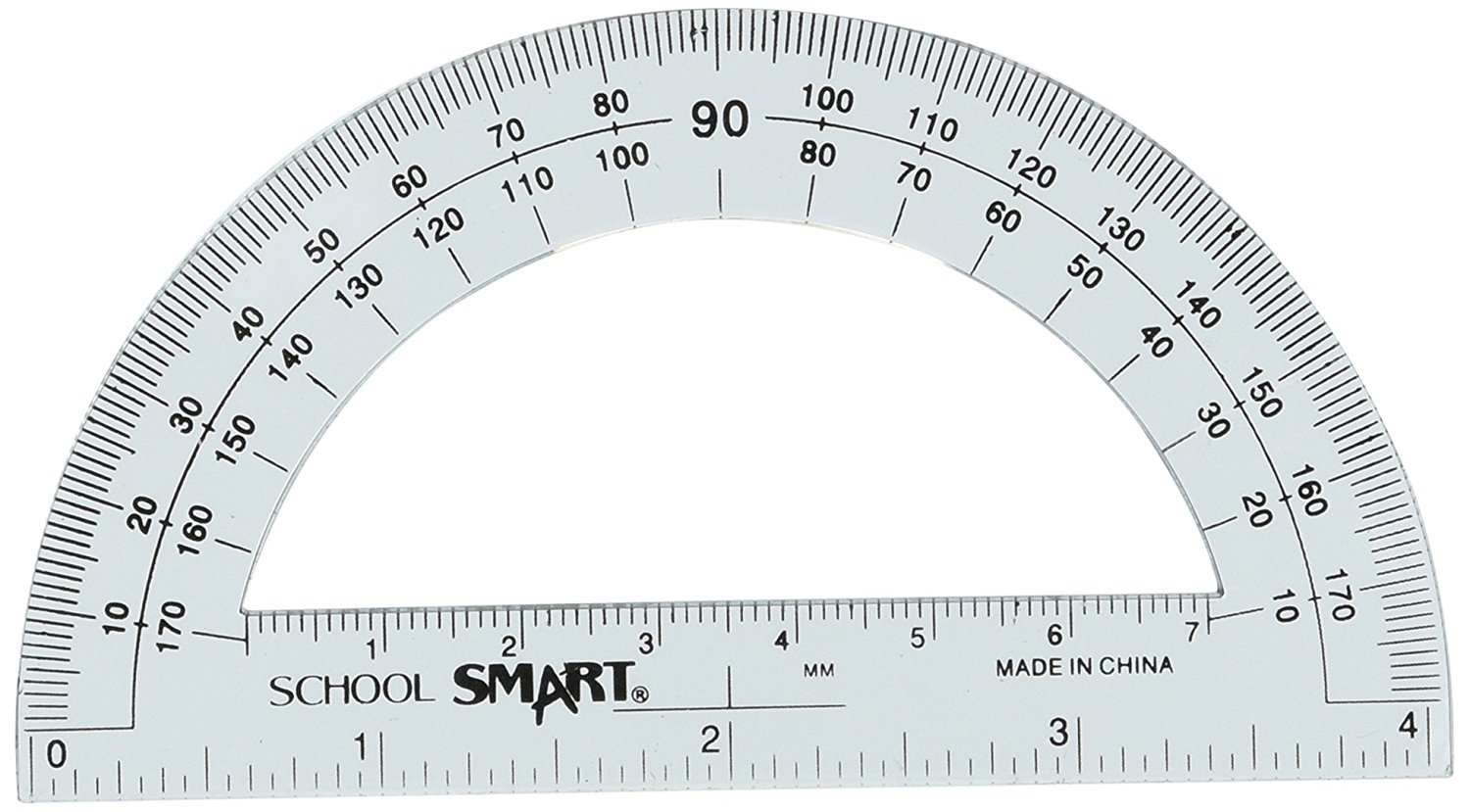23
23
10
Vocabulary Review: What is the name of the tool shown here? Enter a one-word response.
24
10
Vocabulary Review: Draw a line segment on the protractor to represent each angle measure. Then label the angle measures and classify each as acute, right, or obtuse.25
26
27
25
5
Use Your Vocabulary: Does the pair of figures show a rotation? Hint: this refers to the entire figure.
Yes
No
26
5
Use Your Vocabulary: Does the pair of figures show a rotation?
Yes
No
27
5
Use Your Vocabulary: Does the pair of figures show a rotation?
Yes
No
28
10
Reflection: Math Success